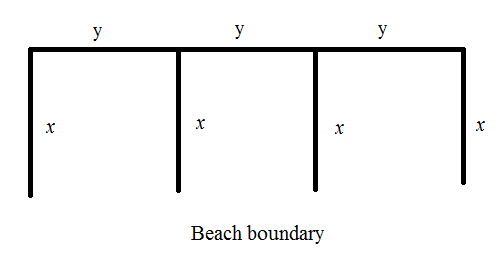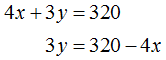# A summer camp has 320320 ft of float line with which to rope off three adjacent rectangular areas of a lake for swimming​ lessons, one for each of three levels of swimming ability. A beach forms one side of the swimming areas. Suppose that the width of each area is x feet. Answer parts​ a) and​ b)  ​a) Express the total area of the three swimming areas as a function of x. ​A(x)equals=​(Simplify your​ answer.) ​b) Find the domain of the function.​(Type your answer in interval​ notation.)

Question
56 views
A summer camp has 320320 ft of float line with which to rope off three adjacent rectangular areas of a lake for swimming​ lessons, one for each of three levels of swimming ability. A beach forms one side of the swimming areas. Suppose that the width of each area is x feet. Answer parts​ a) and​ b)

​a) Express the total area of the three swimming areas as a function of x.

​A(x)equals=

​b) Find the domain of the function.
check_circle

Step 1

Consider the total length of the rope is 320 ft

This rope is used to cover the length of width of rectangle shape swimming area.

Step 2

The rectangle swimming pool are shown in the below figure having one side covered with beach. Consider x ft, y ft be the width and length of each rectangle area.Step 3

The perimeter of the above figure must be equal to 320 ft...

### Want to see the full answer?

See Solution

#### Want to see this answer and more?

Solutions are written by subject experts who are available 24/7. Questions are typically answered within 1 hour.*

See Solution
*Response times may vary by subject and question.
Tagged in

### Trigonometry